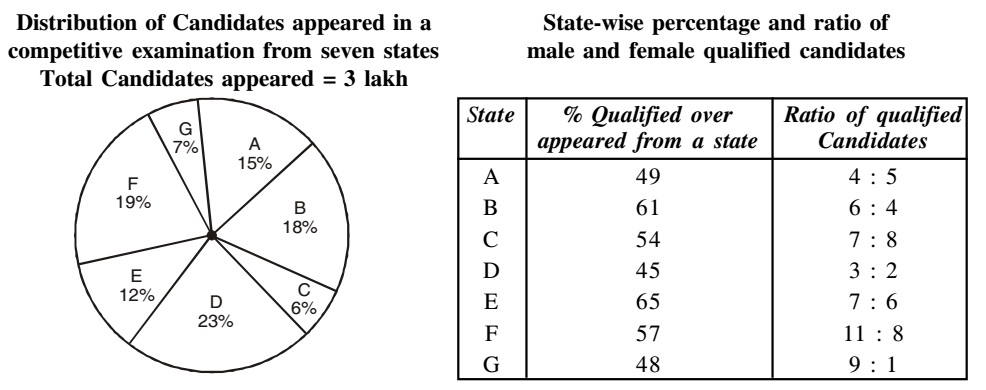Home » Data Interpretation » Mixed Graph » Question

#### Data Interpretation

Direction: Study the following graph and table carefully and answer the questions given below it.1. What is the percentage of candidates qualified from states 'A' and 'C' together of the total candidates appeared?
1. 16.23
2. 18.33
3. 22.23
4. 25.33
##### Correct Option: B

Number of Candidates qualified from States A and C together
= 49/100 x 15/100 x 300000 + 61/100 x 18/100 x 300000
= 22050 + 32940 = 54990
Required percentage = 54990/300000 x 100 = 18.33%